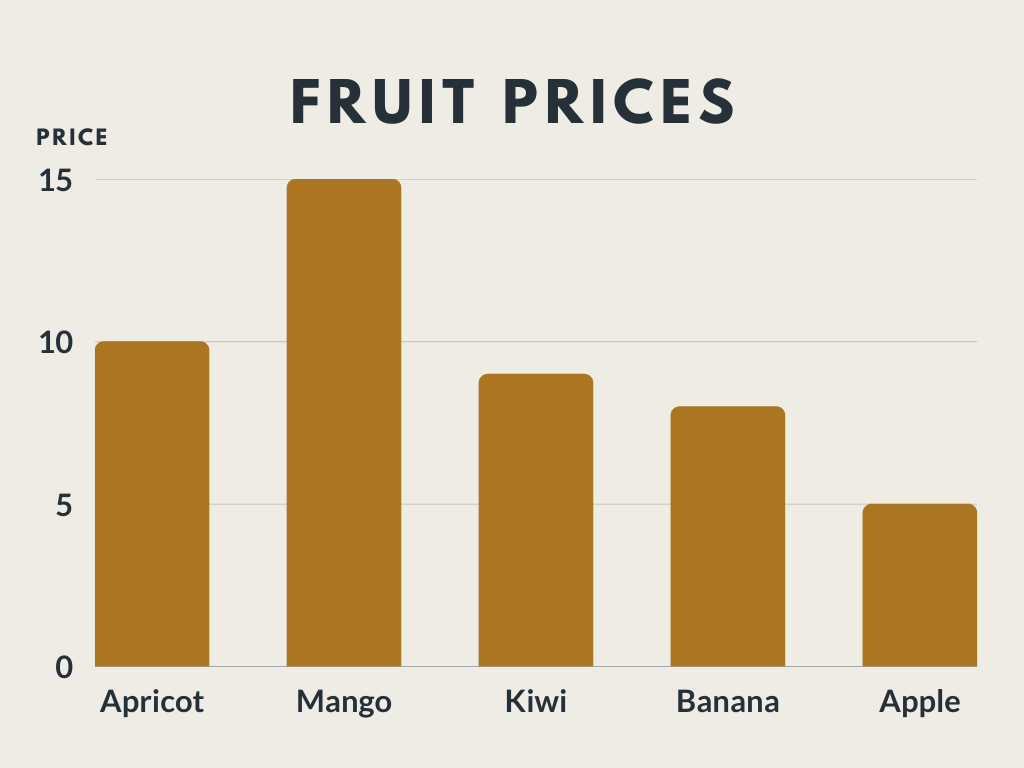Course Content

# First Dive into seaborn Visualization

First Dive into seaborn Visualization

##Countplot

A countplot is a plot that takes a categorical list and returns columns that represent the number of list entries for each category.

An example of a countplot:To initialize a countplot, we need to input at least 1 parameter: `x` (the column in which values will be counted).

Note

We may also use `y` instead of `x` to change the orientation of the plot.

As the `seaborn` is based on the `matplotlib`, we may use a special `matplotlib.pyplot` library and its function `plt.show()` to show the plot:

Look at the code below!Let's solve this problem!1. Import the `seaborn` library with the `sns` alias.
2. Import the `matplotlib.pyplot` library with the `plt` alias.
3. Initialize the x-oriented countplot for `guests_list`.